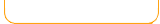Factors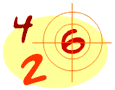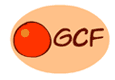Multiples Frenzy
2,3,4,5,6,7,8,9,10,11,12
Multiplication Station
Easy
Multiplication Station
Hard
Greatest Common Factor Least Common Multiple Prime Numbers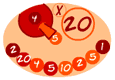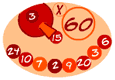Math Lines Math Lines Math Lines Math Lines Math Lines

 What is a factor? Factors are numbers that are multiplied together to get another number. When you factor a number, you are taking the numbers apart and finding the indiviual numbers (factors) that are multiplied within it. Example: What are the factors of the number 6? They are 2 and 3, because 2 x 3 = 6. Also, factors of 6 are 6 and 1, because 6 x 1 = 6. Note: All numbers have the factor of 1, because 1 multiplied by a number equals the same number. So, the factors of 6 are: 1, 2, 3, 6. Example: What are the factors of the number 12? What numbers can be multiplied to get 12? 3 x 4 = 12 2 x 6 = 12 1 x 12 - 12 So the factors of 12 are 1, 2, 3, 4, 6, 12 Example: What are the factors for the number 16? 1 x 16 = 16 2 x 8 = 16 4 x 4 = 16 So the factors of 16 are 1, 2, 4, 8, 16 Prime Numbers A prime number has exactly two factors - the number 1 and itself. It does not have any other factors. Here are some example of prime numbers: Example: the number 7 The only numbers that can divide evenly into 7 are 1 and 7. So 7 is a prime number. Other prime numbers: 2,3,5,7,11,13,17,19,23 ... and the list goes on.